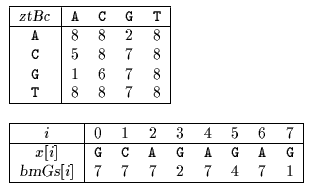Next: Berry-Ravindran Up: ESMAJ Prev: Tuned Boyer-Moore

# Zhu-Takaoka algorithm

Main features
• variant of the Boyer-Moore algorithm;
• uses two consecutive text characters to compute the bad-character shift;
• preprocessing phase in O(m+2) time and space complexity;
• searching phase in O(mn) time complexity.
Description

Zhu and Takaoka designed an algorithm which performs the shift by considering the bad-character shift (see chapter Boyer-Moore algorithm) for two consecutive text characters.

During the searching phase the comparisons are performed from right to left and when the window is positioned on the text factor y[j .. j+m-1] and a mismatch occurs between x[m-k] and y[j+m-k] while x[m-k+1 .. m-1]=y[j+m-k+1 .. j+m-1] the shift is performed with the bad-character shift for text characters y[j+m-2] and y[j+m-1]. The good-suffix shift table is also used to compute the shifts.

The preprocessing phase of the algorithm consists in computing for each pair of characters (a, b) with a, b inthe rightmost occurrence of ab in x[0 .. m-2].

For a,b in: ztBc[a, b]=k iffk
ork=m-1 and x=b and ab does not occur in x[0 .. m-2]
ork=m and xb and ab does not occur in x[0 .. m-2]

It also consists in computing the table bmGs (see chapter Boyer-Moore algorithm).

The preprocessing phase is in O(m+2) time and space complexity. The searching phase has a quadratic worst case.

The C code

The function preBmGs is given chapter Boyer-Moore algorithm.

``` void preZtBc(char *x, int m, int ztBc[ASIZE][ASIZE]) {
int i, j;

for (i = 0; i < ASIZE; ++i)
for (j = 0; j < ASIZE; ++j)
ztBc[i][j] = m;
for (i = 0; i < ASIZE; ++i)
ztBc[i][x] = m - 1;
for (i = 1; i < m - 1; ++i)
ztBc[x[i - 1]][x[i]] = m - 1 - i;
}

void ZT(char *x, int m, char *y, int n) {
int i, j, ztBc[ASIZE][ASIZE], bmGs[XSIZE];

/* Preprocessing */
preZtBc(x, m, ztBc);
preBmGs(x, m, bmGs);

/* Searching */
j = 0;
while (j <= n - m) {
i = m - 1;
while (i < m && x[i] == y[i + j])
--i;
if (i < 0) {
OUTPUT(j);
j += bmGs;
}
else
j += MAX(bmGs[i],
ztBc[y[j + m - 2]][y[j + m - 1]]);
}
}

```
The example

Preprocessing phaseztBc and bmGs tables used by Zhu-Takaoka algorithm.

Searching phase

References
• ZHU R.F., TAKAOKA T., 1987, On improving the average case of the Boyer-Moore string matching algorithm, Journal of Information Processing 10(3):173-177.Next: Berry-Ravindran Up: ESMAJ Prev: Tuned Boyer-Moore

e-mails: {Christian.Charras, Thierry.Lecroq }@laposte.net
Tue Jan 14 15:03:31 MET 1997